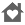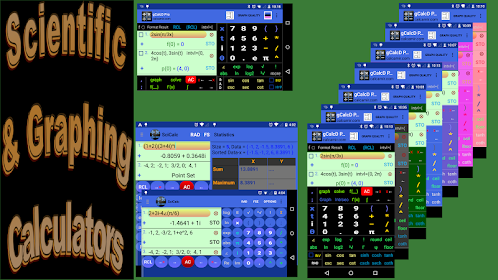# Scientific Calculator | Complex Number CalculatorEveryone
78
This scientific calculator handles complex numbers in a natural way. Any operation or function evaluation you can do with real numbers, you can also do the same with complex numbers by using this scientific calculator. Namely, calculate expressions containing real, imaginary and, in general, any complex number in any form including, but not limited to, the standard (rectangular) and polar forms.

You can enter complex numbers in the standard (rectangular) or in the polar form. The calculated values will be displayed in standard form; optionally, this scientific calculator displays the results in the polar form and other modular forms.

With a unique intuitive user interface this scientific calculator, being a comprehensive complex number calculator, computes arbitrary complicated complex number expressions with exceptional ease.

• Supports all standard mathematical functions with real, imaginary and complex numbers as their arguments including trigonometric, exponential, logarithmic, hyperbolic and inverse trig/hyperbolic functions plus the Gamma function, Γ, and Psi function, Ψ.

Swipe the number pad to the right to see more functions (trig, hyperbolic, inverses, etc.).

• Calculate expressions containing complex numbers in standard form a+bi and polar (phasor) form r∠(θ).

• Convert complex numbers from rectangular to polar and other modular forms and vice versa (long press i or ∠ to see all forms of the calculated result).

• RAD and DEG mode.
In addition to evaluating trig and related functions, this scientific complex number calculator allows the angle of polar form of complex numbers to be entered in radians or degrees.

• Fixed, scientific and engineering notations.

This scientific calculator also let you do statistics with one or two dimensional real data:
• x1, x2 ,x3, ... .
• Press Σ to calculate sum, mean, max, min, variance, sample and standard deviation, median, upper and lower quartile, etc.

• x1, y1; x2, y2; x3, y3; ... .
• Press Σ to calculate the equation of the linear regression line and graph it.

• Long press ! to calculate combinations C(n, r) and permutations P(n, r).

• Enter functions f(x) or parametric equations p(t) and generate table of values.

• Physical/Chemical/Atomic and other scientific constants.

• Easy to use unit converter (Time, Mass, Length, Velocity, and many more).

Use this complex number calculator as a full scientific calculator to evaluate mathematical expressions containing real, imaginary and, in general, any complex numbers, and do more.

All of the above with more details are included in the built-in Instruction menu accompanying this scientific complex calculator.
Collapse

Review Policy
4.4
78 total
5
4
3
2
1

## What's New

Performance improvement.
Collapse

Eligible for Family LibraryLearn More
Updated
March 8, 2019
Size
1.3M
Installs
10,000+
Current Version
1.2.3
Requires Android
4.0.3 and up
Content Rating
Everyone
Permissions
Offered By
gCalcD.com
Developer
22 wood house rd London n12 0rg